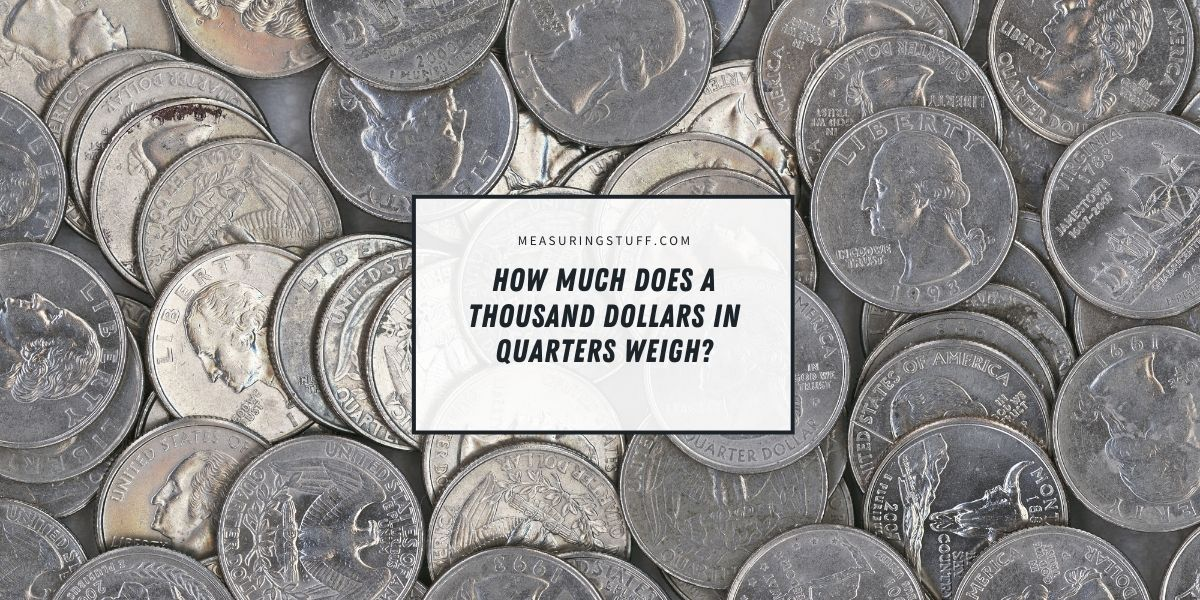# How Much Does A Thousand Dollars In Quarters Weigh?

Last updated on September 3rd, 2023 at 06:51 pm

You only need four quarters to make a dollar, so it might not seem like it would take too many to reach \$1,000.

However, when you consider how many quarters you would need for a large amount of money, it becomes clear that the weight of those quarters would add up quickly.

Using some simple math can help you calculate how much \$1,000 in quarters weighs.

## How much does a quarter weigh?

Before we can find out how much \$1,000 in quarters weighs, we need to know how much a single quarter weighs.

One quarter weighs exactly 5.67 grams, which is equal to about 0.2 ounces.

## How many quarters are in \$1,000?

The next step in finding out how much \$1,000 in quarters weighs is determining how many quarters it would take to make \$1,000.

It takes four quarters to make \$1, and we know that there are 1,000 \$1 amounts in \$1,000.

This means that we only need to multiply 1,000 by four to find that it would take 4,000 quarters to total \$1,000.

## How much does \$1,000 in quarters weigh?

We know that a quarter weighs 0.2 ounces. We can multiply 0.2 by 4,000 to find that 4,000 quarters is equal to 800 ounces.

There are 16 ounces in 1 pound, so we can divide 800 by 16 to find that 4,000 quarters, which is equal to \$1,000, would weigh almost exactly 50 pounds.

If you want to find out how much \$1,000 in quarters weighs in grams, you can either multiply 4,000 by 5.67 or multiply 50 pounds by 453.592, which is how many grams are in a single pound.

CHECK OUT  How Much Money Is 20 Pounds Of 100 Dollar Bills?

## How many rolls of quarters are equal to \$1,000 pounds?

When quarters are rolled to deposit at the bank, each roll contains \$10 or 40 quarters.

This means that you would need 100 rolls to equal the 4,000 quarters in \$1,000.

You can also find this number using math in a different way.

Using a quarter’s weight of 0.2 ounces, we can multiply by 40 and find that a single roll of quarters should weigh about 8 ounces of half a pound, not including the paper wrapper.

We know that \$1,000 in quarters is equal to 50 pounds, so we can divide 50 by 0.5 to get 100, telling us you would need 100 full rolls of quarters.

## How much would \$1,000 in other coins weigh?

If you use the same math formulas as above, substituting the different denomination values and coin weights, you can easily find how much \$1,000 would weigh in any coin denomination.

For example, you would need 100,000 pennies to make up \$1,000. A single penny weighs 2.5 grams or about 0.088 ounces.

This means that \$1,000 in pennies would weigh about 8,800 ounces or around 550 pounds. This is equal to about 250 kilograms.

A nickel, on the other hand, weighs 5 grams or about 0.1764 ounces. One nickel is worth 5 cents, so you need 20 nickels to make up \$1 and 20,000 to add up to \$1,000.

The weight of a nickel multiplied by 20,000 gives us 3,528 ounces or 220.5 pounds. This equals just slightly more than 100 kilograms.

Dimes are the lightest United States coins, weighing only 2.268 grams or 0.08 ounces.

Each dime is worth 10 cents, so you would need 10 dimes to equal \$1 or 10,000 to equal \$1,000.

Multiplying 10,000 by 0.08 ounces gives us 800 ounces or 50 pounds.

This means that \$1,000 in quarters and \$1,000 in dimes weigh the same amount.